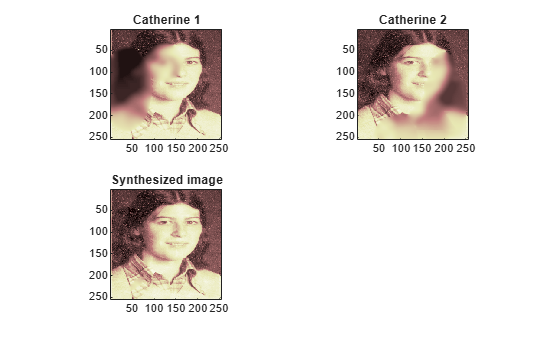# Image Fusion

The principle of image fusion using wavelets is to merge the wavelet decompositions of the two original images using fusion methods applied to approximations coefficients and details coefficients. The two images must be of the same size and are supposed to be associated with indexed images on a common colormap (see wextend to resize images).

Two examples are examined: the first one merges two different images leading to a new image and the second restores an image from two fuzzy versions of an original image.

### Fusion of Two Different Images

```load mask; X1 = X; load bust; X2 = X;```

Merge the two images from wavelet decompositions at level 1 using db2 by taking two different fusion methods: fusion by taking the mean for both approximations and details:

`XFUSmean = wfusimg(X1,X2,'db2',1,'mean','mean');`

and fusion by taking the maximum for approximations and the minimum for the details.

`XFUSmaxmin = wfusimg(X1,X2,'db2',1,'max','min');`

Plot original and synthesized images.

```colormap(map); subplot(221), image(X1), axis square, title('Mask') subplot(222), image(X2), axis square, title('Bust') subplot(223), image(XFUSmean), axis square, title('Synthesized image, mean-mean') subplot(224), image(XFUSmaxmin), axis square, title('Synthesized image, max-min')```### Restoration by Fusion from Fuzzy Images

Load two fuzzy versions of an original image.

```load cathe_1; X1 = X; load cathe_2; X2 = X;```

Merge the two images from wavelet decompositions at level 5 using sym4 by taking the maximum of absolute value of the coefficients for both approximations and details.

`XFUS = wfusimg(X1,X2,'sym4',5,'max','max');`

Plot original and synthesized images.

```figure('Color','white'),colormap(map); subplot(221), image(X1), axis square, title('Catherine 1') subplot(222), image(X2), axis square, title('Catherine 2') subplot(223), image(XFUS), axis square, title('Synthesized image')```##### SupportGet trial now